Courses

# Test: Measurement Of Capacity - 2

## 10 Questions MCQ Test Mathematics (Maths) for Class 3 | Test: Measurement Of Capacity - 2

Description
This mock test of Test: Measurement Of Capacity - 2 for Class 3 helps you for every Class 3 entrance exam. This contains 10 Multiple Choice Questions for Class 3 Test: Measurement Of Capacity - 2 (mcq) to study with solutions a complete question bank. The solved questions answers in this Test: Measurement Of Capacity - 2 quiz give you a good mix of easy questions and tough questions. Class 3 students definitely take this Test: Measurement Of Capacity - 2 exercise for a better result in the exam. You can find other Test: Measurement Of Capacity - 2 extra questions, long questions & short questions for Class 3 on EduRev as well by searching above.
QUESTION: 1

Solution:
QUESTION: 2

Solution:
QUESTION: 3

### Madhavi opened a bottle containing 1 liter of juice. If the shared the juice equally with her uncle Jack. How many milliliters of juice will each of them get?

Solution:
QUESTION: 4

If you want to fill your swimming pool up with water then, which container would you use?

Solution:

Since, swimming pool is very large in capacity so we have to choose the container with maximum capacity out of the given container. As, bucket has maximum capacity out of the given container. Therefore, the answer is option (c) bucket.

QUESTION: 5

If students of class 3 are going for a big picnic then which lemonade should be bought?

Solution:

Since, whole class 3 is going for a picnic to you requires the maximum volume of lemonade for all the students. As 5 liter bottle has maximum volume of lemonade. Therefore, the answer is option (b).

QUESTION: 6

If 2540 ml : 2 It 540 ml ::?: 1 It 5 ml

Solution:

1 It 5 ml = (1000 +5) ml = 1005 ml. Therefore, the answer is option (d) 1005 ml.

QUESTION: 7

If 3 It 690 ml : 369 ml : : ? ; 3020 ml

Solution:

3020 ml = (3000 + 20) ml = 3 It 20 ml. Therefore, the answer is option (a) 3 It 20 ml.

QUESTION: 8

How many buckets of water are needed to fill the water cooler of capacity 45 liter? When the capacity of bucket is 5 Its.

Solution:

Capacity of bucket = 5000 ml = 5 It Capacity of water cooler = 45 It Since, 5 x 9 = 45

∴ Number of buckets of water required to fill the water cooler = 9. Therefore, the answer is option (d) 9.

QUESTION: 9

Chandu is trying to match the objects with their capacity. Match the objects given in List I with List II to help Chandu.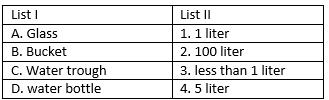Solution:

Capacity of a glass is less than 1 liters so A→ 3. Capacity of a bucket is about 5 liters so B → 4. Capacity of a water trough is about 100 liters so C → 2. Capacity of a water bottle is about 1 liter so D →1. Therefore, the answer is option (b) 3421

QUESTION: 10

Munni has collected some jars. She wants to find the jar which can hold maximum water. Can you find that for Munni?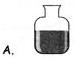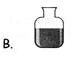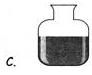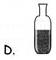Solution:

The Jar C can hold maximum water since it has the maximum capacity among all. Therefore, the answer is option (b) C.